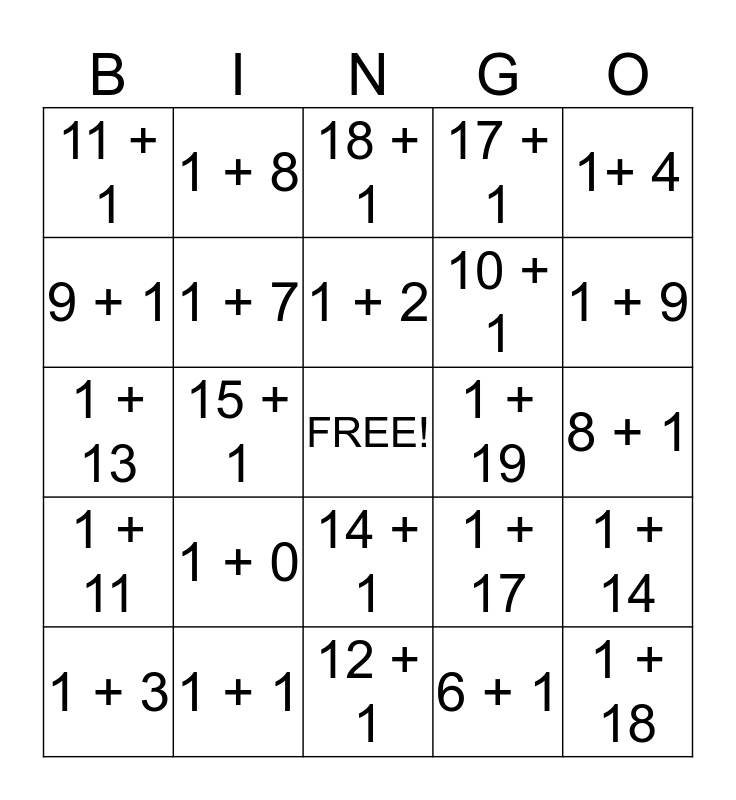# +1 BingoThis bingo card has a free space and 24 words: 1 + 0, 1 + 1, 1 + 2, 1 + 3, 1+ 4, 11 + 1, 9 + 1, 1 + 8, 1 + 19, 14 + 1, 10 + 1, 1 + 13, 1 + 17, 1 + 14, 1 + 7, 6 + 1, 8 + 1, 18 + 1, 1 + 9, 15 + 1, 1 + 18, 1 + 11, 17 + 1 and 12 + 1.

## Play Online